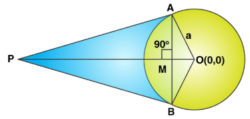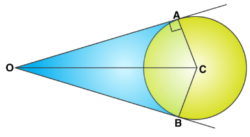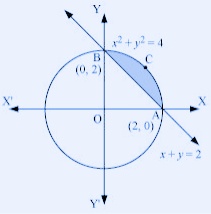Win up to 100% scholarship on Aakash BYJU'S JEE/NEET courses with ABNAT Win up to 100% scholarship on Aakash BYJU'S JEE/NEET courses with ABNAT

# Area Enclosed by Circle

A locus of a point whose distance is constant from the given point is a circle. The centre of the circle is a fixed point, and the fixed distance is the radius. The circumference is the perimeter of the circle. This article explains how to find the area enclosed by circle.

The equation of a circle with radius r and centre (a, b) is given by

(x + a)2 + (y + b)2 = r2

## The Formula for Area Bounded by a Circle

The area enclosed by 2 concentric circles is given by

• If R and r are radii of two concentric circles, then the area enclosed is

$$\begin{array}{l}\pi R^2 – \pi r^2 = \pi(R^2 – r^2) = \pi(R+r)(R-r)\end{array}$$

The method of integration is used to find the areas and volumes by adding up small pieces. A series of concentric rings with radius r where r varies from 0 at the origin to R outside the circle.

2πr gives the circumference, which is the area of each ring.

The formula for finding the area bounded by a circle through integration is given by

$$\begin{array}{l}A= \int_{0}^{R}2\pi r\; dr = 2\pi \times \frac{1}{2}[r^{2}]^{R}_{0} = \pi R^{2}\end{array}$$

## Problems on Area Enclosed by a Circle

Example 1: The area of the triangle formed by the tangents from the points (h, k) to the circle x2 + y2 = a2 and the line joining their points of contact is __________.

Solution:Equation of chord of contact AB is xh + yk = a2 …..(i)

OM = Length of the perpendicular from O (0, 0) on line (i)

$$\begin{array}{l}=\frac{a^2}{\sqrt{h^2+k^2}}\end{array}$$

Therefore, AB = 2 AM

$$\begin{array}{l}= 2 \sqrt{OA^2 – OM^2}\end{array}$$
$$\begin{array}{l}=\frac{2a\sqrt{h^2 + k^2 – a^2}}{\sqrt{h^2+k^2}}\end{array}$$

Also, PM = Length of the perpendicular from P (h, k) to the line (i)

$$\begin{array}{l}=\frac{\sqrt{h^2 + k^2 – a^2}}{\sqrt{h^2+k^2}}\end{array}$$

Therefore, the required area of triangle PAB = [1 / 2] * AB * PM

$$\begin{array}{l}=\frac{a (h^2 + k^2 – a^2)^{\frac{3}{2}}}{(h^2+k^2)}\end{array}$$

Example 2: If OA and OB are the tangents from the origin to the circle x2 + y2 + 2gx + 2fy + c = 0 and C is the centre of the circle, the area of the quadrilateral OACB is _________.

Solution:Area of quadrilateral = 2 [area of ΔOAC]

$$\begin{array}{l}=2 \times \frac{1}{2} \times OA \times AC\end{array}$$
$$\begin{array}{l}=\sqrt{S_1} \times \sqrt{g^2+f^2-c}\end{array}$$

Point is (0, 0) ⇒ S1 = c.

∴ Area = √c * √[g2 + f2 − c]

Example 3: The equation of the tangent to the circle x2 + y2 = a2, which makes a triangle of area a2 with the co-ordinate axes, is __________.

Solution:

Let the tangent be of the form [x / x1] + [y / y1] = 1, and the area of Δ formed by it with coordinate axes is

[1 / 2] * x1y1 = a2 …….(i)

Again, y1x + x1y − x1y1 = 0

On applying conditions of tangency

$$\begin{array}{l}|-\frac{x_1 y_1}{\sqrt{x_1^2 + y_1^2}}| = a\end{array}$$

or

[ x21+ y21] = [x21 y21] / [a2] ………. (ii)

From (i) and (ii), we get x1 and y1, which gives the equation of tangent as x ± y = ± a√2.

Trick: There may be 4 tangents. The lines x ± y = ± a√2 make a triangle of the area in all four quadrants.

Example 4: The area of the triangle formed by the tangent at (3, 4) to the circle x2 + y2 = 25, and the co-ordinate axes is ___________.

Solution:

The equation of the tangent at P (3, 4) to the circle x2 + y2 = 25 is 3x + 4y = 25, which meets the coordinate axes at A (25/3, 0) and B (0, 25/4).

If O is the origin, then the ΔOAB is a right-angled triangle with OA = 25/3 and OB = 25/4.

Area of the ΔOAB = (1/2) × OA × OB

= (1/2) × (25/3) × (25/4)

= 625 / 24

Example 5: The lines 2x − 3y = 5 and 3x − 4y = 7 are the diameters of a circle of area 154 square units. The equation of the circle is _________.

Solution:

Centre of circle = Point of intersection of diameters = (1, ­1)

Now, area = 154

⇒ πr2 = 154

⇒ r = 7

Hence, the equation of required circle is (x − 1)2 + (y + 1)2 = 72

⇒ x2 + y2 − 2x + 2y = 47.

Example 6: A circle is concentric with the circle x2 + y2 −6x + 12y + 15 = 0 and has an area double its area. The equation of the circle is given by ___________.

Solution:

Equation of circle concentric to given circle is x2 + y2 −6x + 12y + 15 = 0 ……(i)

The radius of the circle (i) = √2 (Radius of the given circle)

⇒ √[9 + 36 − k] = √2 * √[9 + 36 − 15]

⇒ 45 − k = 60

⇒ k = −15

Hence, the required equation of the circle is x2 + y2 −6x + 12y – 15 = 0.

Example 7: Find the smaller area enclosed by the circle x2 + y2 = 4 and the line x + y = 2.

Solution:

The smaller area enclosed by the circle x2 + y2 = 4 and the line x + y = 2 is represented by the shaded area ACBA asIt can be observed that Area ACBA = Area OACBO – Area (ΔOAB)

$$\begin{array}{l}=\int_0^2 \sqrt{4-x^2} dx – \int_0^2 (2-x) dx\end{array}$$
$$\begin{array}{l}=[\frac{x}{2} \sqrt{4-x^2} + \frac{4}{2} sin^{-1} \frac{x}{2}]_0^2 – [2x – \frac{x^2}{2}]_0^2\end{array}$$
$$\begin{array}{l}=2 \times \frac{\pi}{2} – (4-2)\end{array}$$

= (π – 2) Units

Example 8: Find the area of a circle with radius a.

Solution:

The equation of the circle is given by x 2 + y 2 = a 2

The circle being symmetric about the x and y axes, the area of the circle can be found by multiplying 4 in order to arrive at the total area of the circle.

Solve the above equation for y.

$$\begin{array}{l}y = \sqrt{a^2-x^2}\end{array}$$

The equation of the upper semi-circle (y positive) is given by

$$\begin{array}{l}y = \sqrt{a^2-x^2}=a \sqrt{1 – \frac{x^2}{a^2}}\end{array}$$

Use integrals to find the area of the upper right quarter of the circle as follows:

$$\begin{array}{l}\frac{1}{4}\cdot \text{Area of circle} = \int_0^a a \sqrt{\frac{1-x^2}{a^2}} dx\end{array}$$

Substitute x / a by sin t so that sin t = x / a and dx = a cos t dt, and the area is given by

$$\begin{array}{l}\frac{1}{4}\cdot \text{Area of circle} =\int_0^{\frac{\pi}{2}} a^2 (1- sin^2 t) cos\ t\ dt\end{array}$$

Now, use the trigonometric identity.

$$\begin{array}{l}\sqrt{ 1 – sin^2 t }= cos t ;\end{array}$$
since t varies from 0 to π/2, hence

$$\begin{array}{l}\frac{1}{4}\cdot \text{Area of circle}= \int_0^{\frac{\pi}{2}} a^2 cos^2 t\ dt\end{array}$$

Use the trigonometric identity cos2 t = ( cos 2t + 1 ) / 2 to linearise the integrand;

$$\begin{array}{l} \frac{1}{4}\cdot \text{Area of circle} = \int_0^{\frac{\pi}{2}} a^2 \frac{(cos\ 2 t + 1)}{2} dt\end{array}$$

Evaluate the integral.

(1 / 4) Area of circle = (1/2) a 2 [ (1/2) sin 2t + t ]0π/2

= (1/4) π a 2

The total area of the circle is obtained through multiplication by 4.

Area of circle = 4 * (1/4) π a 2 = π a 2

Example 9: The area of the region, enclosed by the circle x2 + y2 = 2, which is not common to the region bounded by the parabola y2 = x and the straight line y = x, is

Solution:

The equation of the circle is: x2 + y2 = 2

The required area is

$$\begin{array}{l}=\pi \times (\sqrt{2})^{2}-\int_{0}^{1}(\sqrt{x}-x)dx\\ =2 \pi – [\frac{2}{3}x^{\frac{3}{2}}-\frac{1}{2}x^{2}] \text{ limits from 0 to 1} \\ =2 \pi – [\frac{2}{3}-\frac{1}{2}]\\ =2 \pi – [\frac{1}{6}]\\ =\frac{12 \pi – 1}{6} ​\end{array}$$

Example 10: What is the area bounded by the circle x2 + y2 = 8, the parabola x2 = 2y and the line y = x in y ≥ 0?

Solution:

Required area

= ∫-22 √(8-x2) dx – ∫-20 ½ x2 dx – ∫02 x dx

= 2∫02√(8-x2) dx – [x3/6]-20 – [x2/2]02

= 2[(x/2)√(8-x2) + (8/2)sin-1(x/2√2)]02 – (4/3) – 2

= 2[2 + 4.π/4] – 10/3

= (⅔) + 2π

Example 11: What is the area bounded by the circles x + y2 = r2, r = 1, 2 and the rays 2(x)2 – 3xy – 2(y)2 = 0?

Solution:

2(x)2 – 3xy – 2(y)2 = 0

2(x)2 – 4xy + xy – 2(y)2 = 0

2x(x – 2y) + y(x – 2y) = 0

(2x + y) (x – 2y) = 0

y = -2x or y = x/2

When the two circles of radius 1 and 2 are drawn, the circle with radius 2 is bigger than the circle with radius 1. Now, we have two lines, y = -2x and y = x/2. The slope of the line y = -2x is negative and passes through the origin. Similarly, the slope of the line y = x/2 is found.

Hence, the slope of the line y = -2x is m = -2, and y = x/2 is -1 / 2.

m1m2 = -1

So, the lines are perpendicular.

The required area is

A = π(22)/4 – π(1)2/4

= π – π/4

= 3π/4

Q1

### Give the formula for the area enclosed by two concentric circles.

If R and r are radii of two concentric circles, then the area enclosed is given by the formula π(R2 – r2).

Q2

### What is the area enclosed by a circle of radius r?

Area of the circle = πr2, where r is the radius.

Q3

### What is the difference between the circumference and the area of a circle?

The space enclosed by a circle is called the area. The length of the curved surface is called the circumference of the circle. The area of the circle is πr2, whereas the circumference is given by 2πr (radius is r).

Test your Knowledge on area enclosed by circle